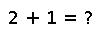# mapslices

mapslices(f, A, dims)

Transform the given dimensions of array `A` using function `f`. `f` is called on each slice of `A` of the form `A[...,:,...,:,...]`. `dims` is an integer vector specifying where the colons go in this expression. The results are concatenated along the remaining dimensions. For example, if `dims` is `[1,2]` and `A` is 4-dimensional, `f` is called on `A[:,:,i,j]` for all `i` and `j`.

## Examples

Checking you are not a robot: